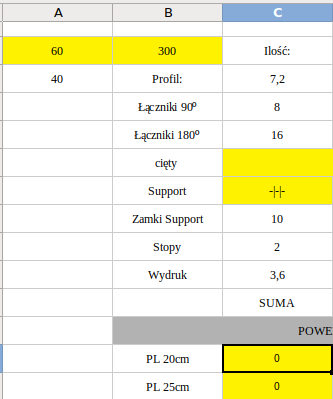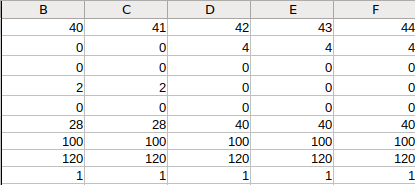# Too many IFs

Hey There,

I need to find out how can I easily make a formula that will input value from the second sheet. At the moment I have it done the way below, however I need to make 300+ IFs this way… Is there a simplier way?
=IF(\$A\$3=\$LED.\$B\$1;\$LED.B2; IF(\$A\$3=\$LED.\$C\$1;\$LED.C2; IFifs.xlsx

It would probably be easier to understand your problem if you were to upload an actual spreadsheet rather than images; however perhaps you might find VLOOKUP more useful than a string of IF statements??

Try to just get rid of the blank cells in the first sheet, fill them with data. After that, the search will become simple and easy - you do not need IF(), most likely SUMPRODUCT() alone will be enough.

Yes, you’re right. I have attached the file. It’s a calculator which is supposed to input automatically a number of LED stripes needed for a certain size. number for LED stripes are prepared in the worksheet ‘LED’. Now, if A3 equals LED.1:1 then it should put in the value of the third row. For exmple if A3 equals 45(which is LED.G1), then the value should be inserted from the LED.G2.

Excuse me a poor explanation, but I am quite new in the excel/calc.

This might work:
`=HLOOKUP( \$A\$3 ; \$LED.\$B\$1:\$JB\$2 ; 2 ; 1 )`

Thank you kind sir!